# GCSE Maths Paper Three (Higher) Practice Paper in the Style of AQA - Calculator

In this assessment, students will be able to complete a timed GCSE Mathematics Paper 3 (Calculator) in the style of AQA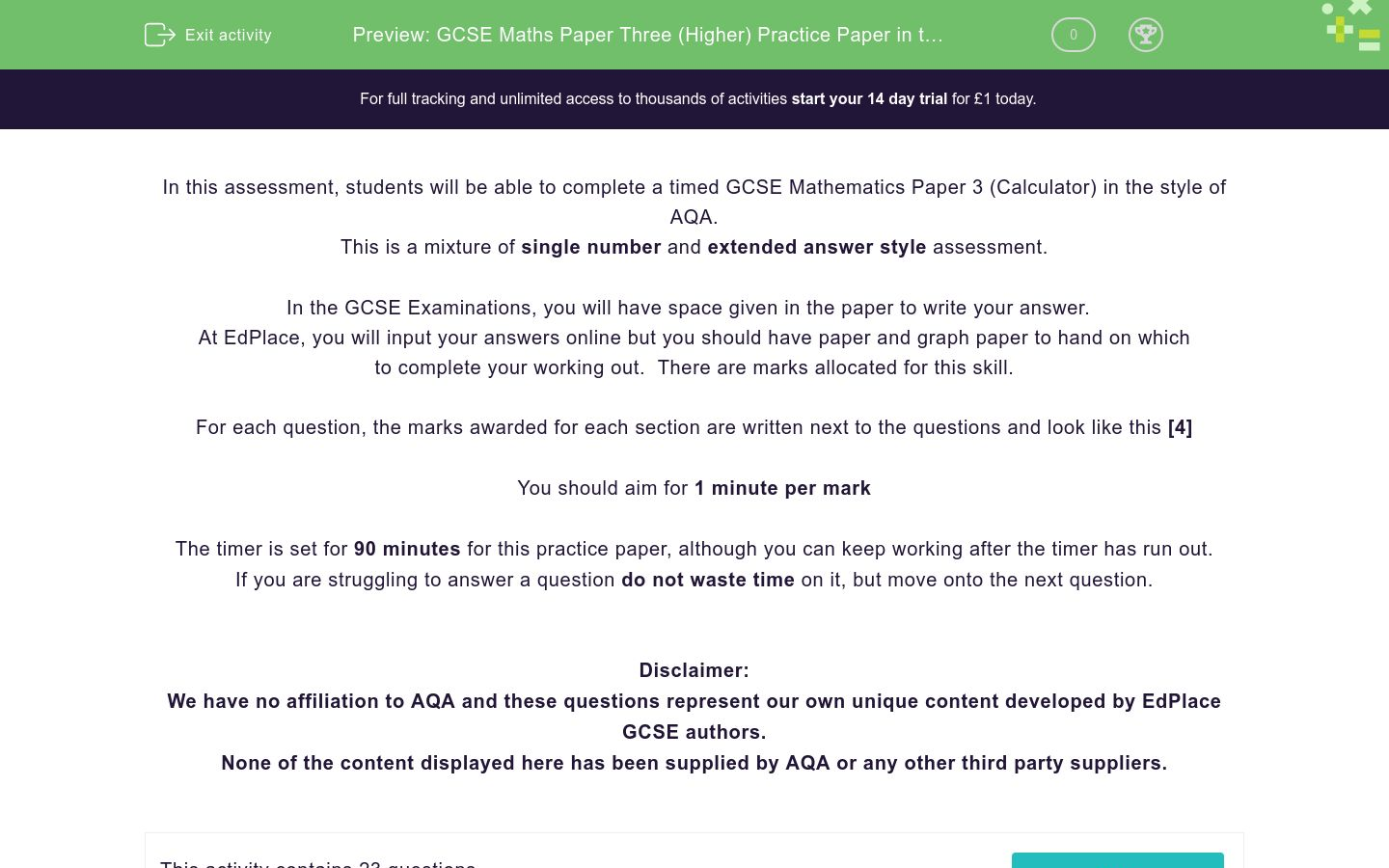Key stage:  KS 4

GCSE Subjects:   Maths

GCSE Boards:   AQA

Curriculum topic:   Higher Practice Papers

Curriculum subtopic:   Calculator Practice Papers

Difficulty level:### QUESTION 1 of 10

In this assessment, students will be able to complete a timed GCSE Mathematics Paper 3 (Calculator) in the style of AQA.

This is a mixture of single number and extended answer style assessment.

In the GCSE Examinations, you will have space given in the paper to write your answer.

At EdPlace, you will input your answers online but you should have paper and graph paper to hand on which to complete your working out.  There are marks allocated for this skill.

For each question, the marks awarded for each section are written next to the questions and look like this 

You should aim for 1 minute per mark

The timer is set for 90 minutes for this practice paper, although you can keep working after the timer has run out.

If you are struggling to answer a question do not waste time on it, but move onto the next question.

Disclaimer:

We have no affiliation to AQA and these questions represent our own unique content developed by EdPlace GCSE authors.

None of the content displayed here has been supplied by AQA or any other third party suppliers.

Solve the simultaneous equations

3x + 2y = 9

4x – 3y = 3.5



(a) Complete this table of values for y = x2 + 2x – 1       

 x -4 -3 -2 -1 0 1 2 y 7 -1 2

(b) On the grid, draw the graph of y = x2 + 2x – 1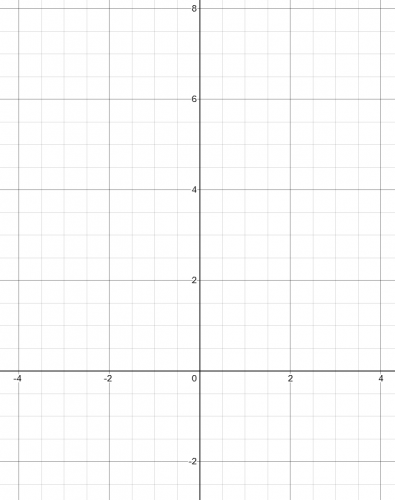(c) Use the graph to estimate a solution to x2 +2x – 1 = 0     

The diagram shows a rectangle ABCE and a triangle ADE

AB = 40 cm

BC = 15 cm.ED:DC = 2:3

Work out the length of the line AD.



Prove that the difference between the squares of any two consecutive numbers is always an odd number.



A motorbike track consists of:

Two straight sections of length 1.8 km and 3 km

Two semicirclesThe length of a motorbike race must be at least 110 km in length.

How many full laps must the race be held over at this circuit?



SolveA hemisphere has a radius of 3x

A cone has a radius of 2x and a height of y

The sphere and the cone have the same volume

Work out the height of the cone: radius of the cone in the form a:b where a and b are integers.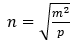m = 3.2 x 105

p = 4.3 x 104

(a) Find the value of n.



m is decreased by 5%

p is decreased by 15%

John says ‘n must be smaller because both m and p are smaller’

(b) Is John correct?



Three toy cars go around a track.

Car A completes a circuit every 40 seconds

Car B completes a circuit every 65 seconds

Car C completes a circuit every 70 seconds.

All three cars start at the same time from the start line.

Will all three cars cross the start line together within one hour?



Here is a pyramid with a square base ABCD.AB = 6 cm

The angle TCA = 41

Find the volume of the pyramid



Here are two similar tins of paintThe volume of tin A: the volume of tin B = 4:3

The surface area of the label on tin B is 100 cm2

Work out the surface area of the label on tin A.



Simplify the following fraction…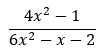Rearrangeto make x the subject.



The graph shows the speed of a coach, in metres per second during the first 20 seconds of a journey.(a) Work out an estimate of the distance travelled in the first 20 seconds. Use 5 strips of equal width.    

(b) Work out the acceleration of the coach in the first 4 seconds.The area of this triangle is (10√3) m2

Calculate the value of X, giving your answer to 3 standard figures.



Solve 2x2 – 3x – 9 < 0



(a) Show that the equation x3 + 2x = 2 has a solution between 0 and 1.      

(b) Show that the equation x3 + 2x = 2 can be rearranged to give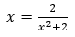(c) Starting with x0 = 0.5,

Use the iteration formula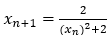three times to find an estimate for the solution of x3 + 2x = 2        

The circle x2 + 3y2 = 10 and the straight line 2x + 3y = 7 intersect at the points A and B.

Find the coordinates of A and B



Here are the first five terms of a quadratic sequence

10    21    38   61    90

Find an expression, in terms of n, for the nth term of this sequence



A shape is translated by the vectorIn which direction does the shape move?



Up

Down

Left

Right

The first four terms of a linear sequence are

2   5   8   11

Select the expression for the nth term.



3n -1

3n +1

n+3

3 - n

What is 1 kg as a fraction of 300 g in it's simplest form?



3/10

10/3

3/7

7/3

Which two of these are the correct solutions to (x + 1)(x - 2) = 0?



x = 2

x = 1

x = -2

x = -1

• Question 1

Solve the simultaneous equations

3x + 2y = 9

4x – 3y = 3.5



EDDIE SAYS• Question 2

(a) Complete this table of values for y = x2 + 2x – 1       

 x -4 -3 -2 -1 0 1 2 y 7 -1 2

(b) On the grid, draw the graph of y = x2 + 2x – 1(c) Use the graph to estimate a solution to x2 +2x – 1 = 0     

EDDIE SAYS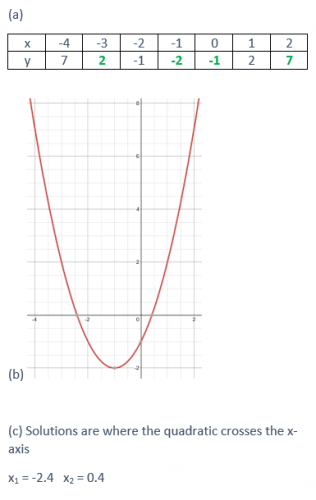• Question 3

The diagram shows a rectangle ABCE and a triangle ADE

AB = 40 cm

BC = 15 cm.ED:DC = 2:3

Work out the length of the line AD.



EDDIE SAYS• Question 4

Prove that the difference between the squares of any two consecutive numbers is always an odd number.



EDDIE SAYS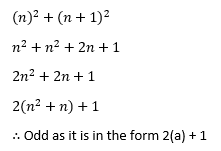• Question 5

A motorbike track consists of:

Two straight sections of length 1.8 km and 3 km

Two semicirclesThe length of a motorbike race must be at least 110 km in length.

How many full laps must the race be held over at this circuit?



EDDIE SAYS• Question 6

SolveEDDIE SAYS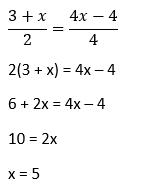• Question 7A hemisphere has a radius of 3x

A cone has a radius of 2x and a height of y

The sphere and the cone have the same volume

Work out the height of the cone: radius of the cone in the form a:b where a and b are integers.



EDDIE SAYS• Question 8m = 3.2 x 105

p = 4.3 x 104

(a) Find the value of n.



m is decreased by 5%

p is decreased by 15%

John says ‘n must be smaller because both m and p are smaller’

(b) Is John correct?



EDDIE SAYS• Question 9

Three toy cars go around a track.

Car A completes a circuit every 40 seconds

Car B completes a circuit every 65 seconds

Car C completes a circuit every 70 seconds.

All three cars start at the same time from the start line.

Will all three cars cross the start line together within one hour?



EDDIE SAYS• Question 10

Here is a pyramid with a square base ABCD.AB = 6 cm

The angle TCA = 41

Find the volume of the pyramid



EDDIE SAYS• Question 11

Here are two similar tins of paintThe volume of tin A: the volume of tin B = 4:3

The surface area of the label on tin B is 100 cm2

Work out the surface area of the label on tin A.



EDDIE SAYS• Question 12

Simplify the following fraction…EDDIE SAYS• Question 13

Rearrangeto make x the subject.



EDDIE SAYS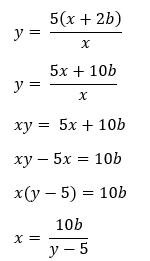• Question 14

The graph shows the speed of a coach, in metres per second during the first 20 seconds of a journey.(a) Work out an estimate of the distance travelled in the first 20 seconds. Use 5 strips of equal width.    

(b) Work out the acceleration of the coach in the first 4 seconds.      

EDDIE SAYS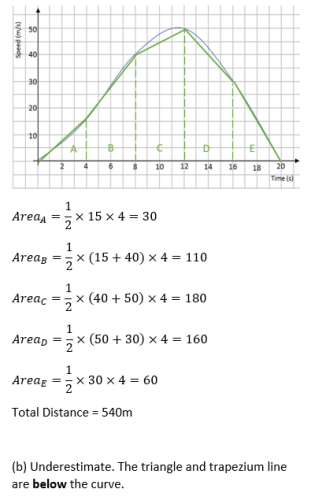• Question 15The area of this triangle is (10√3) m2

Calculate the value of X, giving your answer to 3 standard figures.



EDDIE SAYS• Question 16

Solve 2x2 – 3x – 9 < 0



EDDIE SAYS• Question 17

(a) Show that the equation x3 + 2x = 2 has a solution between 0 and 1.      

(b) Show that the equation x3 + 2x = 2 can be rearranged to give(c) Starting with x0 = 0.5,

Use the iteration formulathree times to find an estimate for the solution of x3 + 2x = 2        

EDDIE SAYS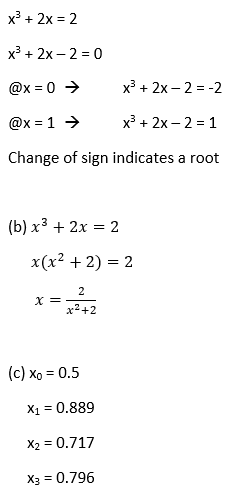• Question 18

The circle x2 + 3y2 = 10 and the straight line 2x + 3y = 7 intersect at the points A and B.

Find the coordinates of A and B



EDDIE SAYS• Question 19

Here are the first five terms of a quadratic sequence

10    21    38   61    90

Find an expression, in terms of n, for the nth term of this sequence



EDDIE SAYS• Question 20

A shape is translated by the vectorIn which direction does the shape move?



Right
EDDIE SAYS
In a translation vector, the top number represents horizontal movement so it can only be left or right. Is a positive number left or right? Think about coordinates.
• Question 21

The first four terms of a linear sequence are

2   5   8   11

Select the expression for the nth term.



3n -1
EDDIE SAYS
All linear sequences follow the form an + b To find a, we look at the difference between the numbers To find b, we ask what number would go before the first term to fit into the pattern,
• Question 22

What is 1 kg as a fraction of 300 g in it's simplest form?



10/3
EDDIE SAYS
To put these into a fraction, we first need to make them the same units. If we change 1 kg into 1000 g. This gives a fraction of 1000/3000 All we have to do to finish is simplify the fraction.
• Question 23

Which two of these are the correct solutions to (x + 1)(x - 2) = 0?



x = 2
x = -1
EDDIE SAYS
When we are solving the equation (x + 1)(x - 2) = 0, we need to remember that there is a little hidden multiply between the brackets. (x + 1) x (x - 2) = 0 If we multiply two brackets together and get zero, this means that one of the brackets must equal zero. So... x + 1 = 0 OR x - 2 = 0 All you need to do is to solve these two simple equations.